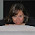## How to

When solving these problems, learning by doing is much better than learning by reading. I encourage to you read only as far in the solution as you need, then trying to solve the problem. If you get stuck, try reading a little further. And of course, let me know if you find a better solution!

## Monday, July 9, 2012

### 0s and 1s oh my!

Problem:  You are given an array with only 1s and 0s.  Sort the array.

void sortArray(int arr[]) {...}

Solution:  Your first thought is probably to use quicksort.  The is a super simple solution and it's worth mentioning:

void sortArray(int arr[]) {
qSort(arr);
}

Well, that was pretty easy and we have a working solution.  We could go ahead and implement quicksort if we had to, but that just shows that we know how to sort and array.  So, let's look at the run-time of this.  Quicksort is O(n log n), so this is an O(n log n) fuction.  Well, can we do better?

At first thought, this is as good as could be as sorting is optimally an O(n log n) function.  But, we do have another piece of information that we haven't used -- which is that the only elements are a 0 or a 1.  Let's make an example array to help us see how to do this.  Figure 1 shows just such an example.

Figure 1

Try looking at figure 1 and see what information it provides.  We see that there are 0s and 1s and a certain number of each.  Try to image what we could do with the certain number of each.

This starts to hint at a possible algorithm.  We can go through and count the number of 0s and 1s.  Then, we can back through the array and write the number of 0s and 1s in place.  This should work, and would be O(n) as we only go through the array twice.

Now, let's code up this algorithm:

void sortArray(int arr[]) {

int curElem, numZeroes = 0;

/* Count the number of zeroes */
for (curElem = 0; curElem < arr.length; curElem++) {
if (arr[curElem] == 0) numZeroes++;
}

/* Now, write all the 0s at the beginning and 1s at the end */
for (curElem = 0; curElem < arr.length; curElem++) {
if (numZeroes > 0) {
arr[curElem] = 0;
numZeroes--;
}  else {
arr[curElem] = 1;
}
}
}

Let's test this with the sample array in figure 1.  numZeroes will count six 0s.  Then, the second for loop will put 6 zeroes at the front until numZeroes equals 0, upon which it will write eight remaining 1s, perfectly sorting the array in linear time.

It's worth testing the boundary cases, like a null array, in which case this would return null, or if there are nonzero characters, which simply become 1s in this case, which seems ok, but we would have to understand in a greater context if there are other ways to handle this.

Pretty neat.  Now, for next time, think of what it would be if we had 1, 2 or 3 in the array.

/* Please let me know if you find alternate solutions or bugs.  Thanks, Noah */

1.The following is a minor optimization, removing a few if statements:

void sortArray(int arr[]) {

int curElem, numOnes = 0;

/* Count the number of zeroes */
for (curElem = 0; curElem < arr.length; curElem++) {
numOnes += arr[curElem];
}

/* Now, write all the 0s at the beginning and 1s at the end */
for (curElem = 0; curElem < arr.length - numOnes; curElem++) {
arr[curElem] = 0;
}
for (; curElem < arr.length; curElem++) {
arr[curElem] = 1;
}
}

2.What about having two indexes in the array? One starts at the first element and increases until we find a 1. The other starts from the end decreasing until finding a zero. When those two things happen then we swap those two elements. We repeat until the indexes reach the same value. At that point the array should be sorted. U traverse the array once. And we need just an additional variable to do the swap.